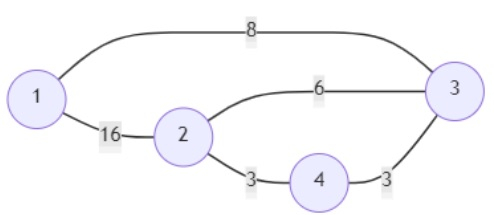# Program to check existence of edge length limited paths in Python

PythonServer Side ProgrammingProgramming

Suppose we have one undirected weighted graph with n nodes using one edgeList, where edgeList[i] has three parameters (u, v, w) denotes there is a path from u to v whose distance is w. We also have another query array where query[i] has (p, q, lim). This query is trying to ask whether there is a path (direct or via some other node) from p to q whose distance is less than lim. We have to return an array holding True/False results for each query.

So, if the input is likethen the output will be [True, False, True]. Because to go from 1 to 4 we can follow path 1 -> 3 - > 4 with cost 11, second one is false because we cannot go from 2 to 3 using less than 3, and last one is true because we can go from 1 to 2 using path 1 -> 3 -> 2 with cost 14 which is less than 15.

To solve this, we will follow these steps −

• parent := a list from 0 to n

• rank := a list of size n+1 and fill with 0

• Define a function find() . This will take parent, x

• if parent[x] is same as x, then

• return x

• parent[x] := find(parent, parent[x])

• return parent[x]

• Define a function union() . This will take parent, a, b

• a := find(parent, a)

• b := find(parent, b)

• if a is same as b, then

• return

• if rank[a] < rank[b], then

• parent[a] := b

• otherwise when rank[a] > rank[b], then

• parent[b] := a

• otherwise,

• parent[b] := a

• rank[a] := rank[a] + 1

• From the main method do the following −

• sort edgeList based on weight parameters

• res := an array with number of queries and fill with 0

• queries := a list of pair (i, ch) for each index i and value ch from queries

• sort queries based on limit parameters

• ind := 0

• for each index i triplet (a, b, w) in queries, do

• while ind < size of edgeList and edgeList[ind, 2] < w, do

• union(parent, edgeList[ind, 0])

• ind := ind + 1

• res[i] := find(parent, a) is same as find(parent, b)

• return res

## Example

Let us see the following implementation to get better understanding

def solve(n, edgeList, queries):
parent = [i for i in range(n+1)]

rank = [0 for i in range(n+1)]

def find(parent, x):

if parent[x] == x:
return x
parent[x] = find(parent, parent[x])
return parent[x]

def union(parent, a, b):

a = find(parent, a)
b = find(parent, b)

if a == b:
return

if rank[a] < rank[b]:
parent[a] = b
elif rank[a] > rank[b]:
parent[b] = a
else:
parent[b] = a
rank[a] += 1

edgeList.sort(key = lambda x: x)
res =  * len(queries)
queries = [[i, ch] for i, ch in enumerate(queries)]
queries.sort(key = lambda x: x)

ind = 0
for i, (a, b, w) in queries:

while ind < len(edgeList) and edgeList[ind] < w:
union(parent, edgeList[ind], edgeList[ind])
ind += 1

res[i] = find(parent, a) == find(parent, b)
return res

n = 4
edgeList = [(1,2,16),(1,3,8),(2,4,3),(2,3,6),(4,3,3),]
queries = [(1,4,12),(2,3,3),(1,2,15)]
print(solve(n, edgeList, queries))

## Input

4, [(1,2,16),(1,3,8),(2,4,3),(2,3,6),(4,3,3)],[(1,4,12),(2,3,3),(1,2,15)]

## Output

[True, False, True]